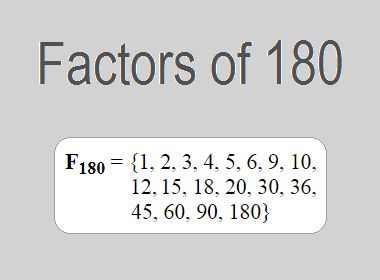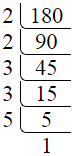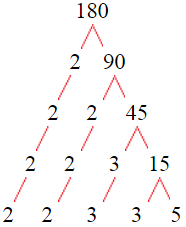# Factors of 180The factors of 180 are 1, 2, 3, 4, 5, 6, 9, 10, 12, 15, 18, 20, 30, 36, 45, 60, 90, and 180 i.e. F180 = {1, 2, 3, 4, 5, 6, 9, 10, 12, 15, 18, 20, 30, 36, 45, 60, 90, 180}. The factors of 180 are all the numbers that can divide 180 without leaving a remainder.

We can check if these numbers are factors of 180 by dividing 180 by each of them. If the result is a whole number, then the number is a factor of 180. Let's do this for each of the numbers listed above:

·        1 is a factor of 180 because 180 divided by 1 is 180.

·        2 is a factor of 180 because 180 divided by 2 is 90.

·        3 is a factor of 180 because 180 divided by 3 is 60.

·        4 is a factor of 180 because 180 divided by 4 is 45.

·        5 is a factor of 180 because 180 divided by 5 is 36.

·        6 is a factor of 180 because 180 divided by 6 is 30.

·        9 is a factor of 180 because 180 divided by 9 is 20.

·        10 is a factor of 180 because 180 divided by 10 is 18.

·        12 is a factor of 180 because 180 divided by 12 is 15.

·        15 is a factor of 180 because 180 divided by 15 is 12.

·        18 is a factor of 180 because 180 divided by 18 is 10.

·        20 is a factor of 180 because 180 divided by 20 is 9.

·        30 is a factor of 180 because 180 divided by 30 is 6.

·        36 is a factor of 180 because 180 divided by 36 is 5.

·        45 is a factor of 180 because 180 divided by 45 is 4.

·        60 is a factor of 180 because 180 divided by 60 is 3.

·        90 is a factor of 180 because 180 divided by 90 is 2.

·        180 is a factor of 180 because 180 divided by 180 is 1.

## How to Find Factors of 180?

1 and the number itself are the factors of every number. So, 1 and 180 are two factors of 180. To find the other factors of 180, we can start by dividing 180 by the numbers between 1 and 180. If we divide 180 by 2, we get a remainder of 0. Therefore, 2 is a factor of 180. If we divide 180 by 3, we get a remainder of 0. Therefore, 3 is a factor of 180.

Next, we can check if 4 is a factor of 180. If we divide 180 by 4, we get a remainder of 0. Therefore, 4 is also a factor of 180. We can continue this process for all the possible factors of 180.

Through this process, we can find that the factors of 180 are 1, 2, 3, 4, 5, 6, 9, 10, 12, 15, 18, 20, 30, 36, 45, 60, 90, and 180. These are the only numbers that can divide 180 without leaving a remainder.

********************

********************

## Properties of the Factors of 180

The factors of 180 have some interesting properties. One of the properties is that the sum of the factors of 180 is equal to 546. We can see this by adding all the factors of 180 together:

1 + 2 + 3 + 4 + 5 + 6 + 9 + 10 + 12 + 15 + 18 + 20 + 30 + 36 + 45 + 60 + 90 + 180 = 546

Another property of the factors of 180 is that the prime factors of 180 are 2, 3, and 5 only.

## Applications of the Factors of 180

The factors of 180 have several applications in mathematics. One of the applications is in finding the highest common factor (HCF) of two or more numbers. The HCF is the largest factor that two or more numbers have in common. For example, to find the HCF of 180 and 120, we need to find the factors of both numbers and identify the largest factor they have in common. The factors of 180 are 1, 2, 3, 4, 5, 6, 9, 10, 12, 15, 18, 20, 30, 36, 45, 60, 90, and 180. The factors of 120 are 1, 2, 3, 4, 5, 6, 8, 10, 12, 15, 20, 24, 30, 40, 60, and 120. The largest factor that they have in common is 60. Therefore, the HCF of 180 and 120 is 60.

Another application of the factors of 180 is in prime factorization. Prime factorization is the process of expressing a number as the product of its prime factors. The prime factors of 180 are 2, 3, and 5, since these are the only prime numbers that can divide 180 without leaving a remainder. Therefore, we can express 180 as:

180 = 2 × 2 × 3 × 3 × 5

We can do prime factorization by division and factor tree method also. Here is the prime factorization of 180 by division method,180 = 2 × 2 × 3 × 3 × 5

Here is the prime factorization of 180 by the factor tree method,180 = 2 × 2 × 3 × 3 × 5

## Conclusion

The factors of 180 are the numbers that can divide 180 without leaving a remainder. The factors of 180 are 1, 2, 3, 4, 5, 6, 9, 10, 12, 15, 18, 20, 30, 36, 45, 60, 90, and 180. The factors of 180 have some interesting properties, such as having a sum of 546. The factors of 180 have several applications in mathematics, such as finding the highest common factor and prime factorization.